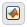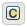## Stateflow 中向量和矩阵的运算

•MATLAB® 作为动作语言。

•C 语言作为动作语言。

### 索引表示法

MATLAB 作为动作语言

C 作为动作语言

`M` 矩阵第 `4` 行第 `5` 列的元素`M(4,5)``M`
`M` 矩阵第 `i` 行第 `j` 列的元素`M(i,j)``M[i-1][j-1]`

MATLAB 作为动作语言

C 作为动作语言

`a * b`

1

`a .* b`

1

`a / b`

1

`a ./ b`

1

`a \ b`

1

`a .\ b`

1

`a + b`

2

`a - b`

2

`a == b`

3

`a ~= b`

3

`a != b`

3

`a <> b`

3

### 一元运算和动作

• 优先于二元运算符。

• 采用右结合，因此在任何表达式中，它们均为从右向左计算。

• 执行按元素运算。

MATLAB 作为动作语言

C 作为动作语言

`~a`

• 按位 NOT（默认值）。通过选中启用 C 语言位运算图属性来启用此运算。

• 逻辑 NOT。通过清除启用 C 语言位运算图属性来启用此运算。

`!a`

`-a`

`a++`

`a--`

### 赋值运算

MATLAB 作为动作语言

C 作为动作语言

`a = b`

`a += b`

`a -= b`

`a *= b`

`a /= b`

MATLAB 作为动作语言

C 作为动作语言

#### 为矩阵的所有元素赋值

`A = [1 2 3; 4 5 6];`

`A = 10;`

`y[i][j] = f(u)`

`y[i][j] = f(u[i][j])`

### 使用 MATLAB 函数执行矩阵算术

• 计算标准矩阵乘积 `y1 = u1 * u2`

• 求解方程 `u1 * y2 = u2`

• 求解方程 `y3 * u1 = u2`

```function [y1, y2, y3] = my_matrix_ops(u1, u2) %#codegen y1 = u1 * u2; % matrix multiplication y2 = u1 \ u2; % matrix division from the right y3 = u1 / u2; % matrix division from the left```